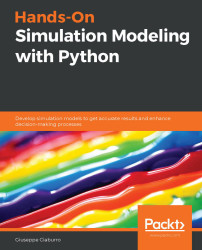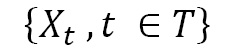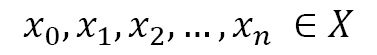•#### Hands-On Simulation Modeling with Python#### Overview of this book

Simulation modeling helps you to create digital prototypes of physical models to analyze how they work and predict their performance in the real world. With this comprehensive guide, you'll understand various computational statistical simulations using Python. Starting with the fundamentals of simulation modeling, you'll understand concepts such as randomness and explore data generating processes, resampling methods, and bootstrapping techniques. You'll then cover key algorithms such as Monte Carlo simulations and Markov decision processes, which are used to develop numerical simulation models, and discover how they can be used to solve real-world problems. As you advance, you'll develop simulation models to help you get accurate results and enhance decision-making processes. Using optimization techniques, you'll learn to modify the performance of a model to improve results and make optimal use of resources. The book will guide you in creating a digital prototype using practical use cases for financial engineering, prototyping project management to improve planning, and simulating physical phenomena using neural networks. By the end of this book, you'll have learned how to construct and deploy simulation models of your own to overcome real-world challenges.
PrefaceSection 1: Getting Started with Numerical SimulationFree Chapter
Chapter 1: Introducing Simulation ModelsChapter 2: Understanding Randomness and Random NumbersChapter 3: Probability and Data Generation ProcessesSection 2: Simulation Modeling Algorithms and TechniquesChapter 4: Exploring Monte Carlo SimulationsChapter 5: Simulation-Based Markov Decision ProcessesChapter 6: Resampling MethodsChapter 7: Using Simulation to Improve and Optimize SystemsSection 3: Real-World ApplicationsChapter 8: Using Simulation Models for Financial EngineeringChapter 9: Simulating Physical Phenomena Using Neural NetworksChapter 10: Modeling and Simulation for Project ManagementChapter 11: What's Next?Other Books You May Enjoy# Stochastic processes

A stochastic process is a family of random variables that depends on a parameter, t. A stochastic process is specified using the following notation:Here, t is a parameter, and T is the set of possible values of t.

Usually, time is indicated by t, so a stochastic process is a family of time-dependent random variables. The variability range of t, that is, the set, T, can be a set of real numbers, possibly coinciding with the entire time axis. But it can also be a discrete set of values.

The random variables, Xt, are defined on the set, X, called the space of states. This can be a continuous set, in which case it is defined as a continuous stochastic process, or a discrete set, in which case it is defined as a discrete stochastic process.

Consider the following elements:This means the values that the random variables, Xt, can take are called system states and represent the possible results of an experiment...